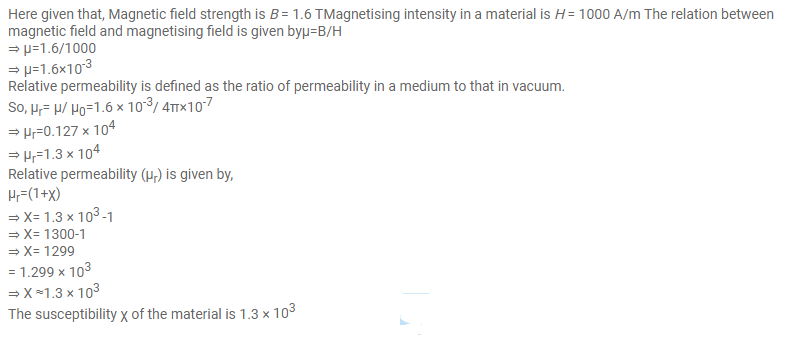# The magnetic field B and the magnetic intensity

Question:

The magnetic field $B$ and the magnetic intensity $\mathrm{H}$ in a material are found to be $1.6 \mathrm{~T}$ and $1000 \mathrm{~A} \mathrm{~m}^{-1}$, respectively. Calculate the relative permeability $\mu_{r}$ and the susceptibility $X$ of the material.

Solution: